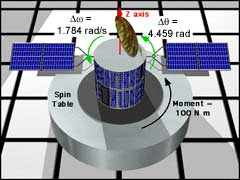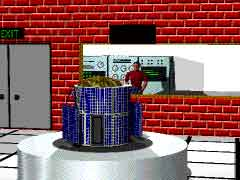Ch 7. Rigid Body Energy Methods Multimedia Engineering Dynamics Rot. Work & Energy Conservation of Energy
 Chapter - Particle - 1. General Motion 2. Force & Accel. 3. Energy 4. Momentum - Rigid Body - 5. General Motion 6. Force & Accel. 7. Energy 8. Momentum 9. 3-D Motion 10. Vibrations Appendix Basic Math Units Basic Equations Sections eBooks Dynamics Fluids Math Mechanics Statics Thermodynamics Author(s): Kurt Gramoll ©Kurt GramollDYNAMICS - CASE STUDY IntroductionProblem DiagramSatellite in Deployed PositionSatellite in Stored Position To accurately control the new satellite, small rocket thrusters will be used to rotate and move the satellite when in orbit. In order to know how long to fire the thrusters, the mass and moment of inertia must be known. Due to the intricate shape of the satellite, you can't accurately calculate the moment of inertia. Your boss tells you to use the spin table to determine the moment of inertia experimentally. What is known: The change in angular displacement Δθ is 4.459 rad. The change in angular velocity Δω is 1.784 rad/s The spin table generates a constant 100 N m torque during the length of the test. The time length of the test was not monitored. Question What is the moment of inertia about the longitudinal axis Iz in the deployed configuration? Approach The acceleration is not known, and thus M = Iα can not be used directly. Use the Principle of Work and Energy for rotating rigid bodies: Work = Change in Kinetic Energy or simply, T1 + ΣU1-2 = T2

Practice Homework and Test problems now available in the 'Eng Dynamics' mobile app
Includes over 400 problems with complete detailed solutions.
Available now at the Google Play Store and Apple App Store.